# OpenCV中轮廓处理简介

在OpenCV中，将 Canny 等边缘检测算法根据像素间的差异检测出轮廓边界的像素，作为一个整体来研究和分析，称之为轮廓。比如对于这副图片：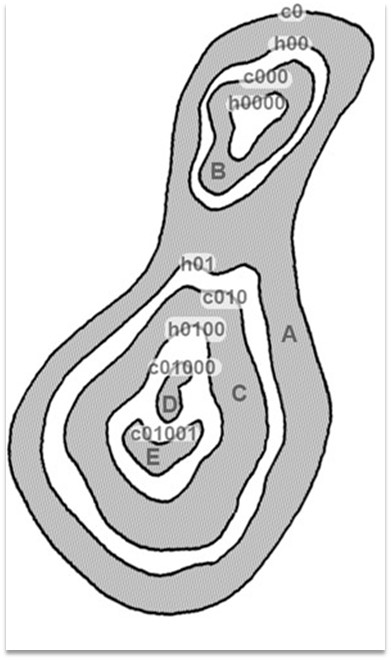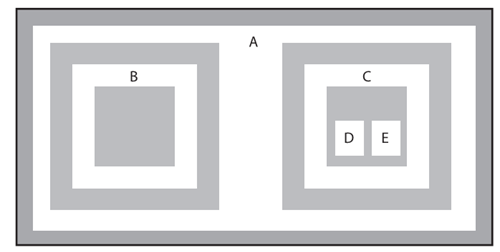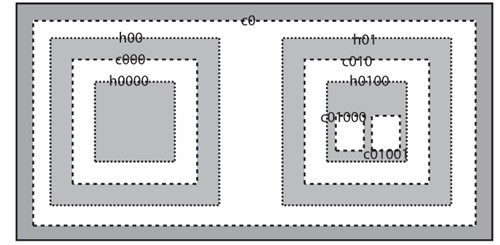void cv::findContours(  cv::InputOutputArray    image,               // 输入图像，特别需要注意是二值图像       cv::OutputArrayOfArrays contours,        //输出结果  cv::OutputArray         hierarchy,              // 层级结果  int                     mode,                             //定义轮廓是如何提取  int                     method,                          // 定义轮廓的寻找方法    cv::Point           offset = cv::Point()     // Offset every point);

参数一：输入图像，8位单通道；参数二：“an array of arrays”，一般采用“ an STL vector of STL vectors”，找到的轮廓、函数调用后的运算结果保存在这里；参数三：hierarchy（层次，等级），可选输出向量。包含图像的拓扑信息。每个轮廓对应4元组，分别对应后一个轮廓、前一个轮廓、父轮廓和内嵌轮廓。参数四：flag 轮廓检索模式参数五：flag 轮廓近似方法

 Index Meaning 0 同级的下一条轮廓 1 同级的前一条轮廓 2 下级的第一个子节点 3 上级的父节点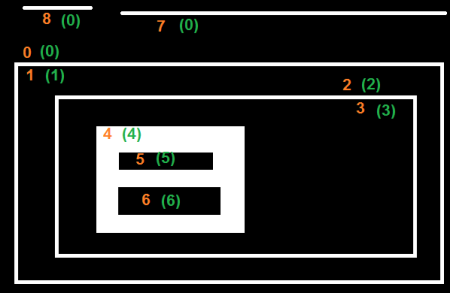0  [ 7, -1, 1, -1]1  [-1, -1, 2, 0]2  [-1, -1, 3, 1]3  [-1, -1, 4, 2]4  [-1, -1, 5, 3]5  [ 6, -1, -1, 4]6  [-1, 5, -1, 4]7  [ 8, 0, -1, -1]8  [-1, 7, -1, -1]

cv::CHAIN_APPROX_NONE

cv::CHAIN_APPROX_SIMPLE

cv::CHAIN_APPROX_TC89_L1 or cv::CHAIN_APPROX_TC89_KCOS

cv::arcLength() 得到轮廓的长度
double  cv::arcLength(  cv::InputArray  points,      // Array or vector of 2-dimensional points  bool            closed       // If true, assume link from last to first vertex);

cv::minAreaRect()活动轮廓的最小外接矩形
cv::RotatedRect cv::minAreaRect( // Return rectangle bounding the points  cv::InputArray  points,        // Array or vector of 2-dimensional points);

cv::minEnclosingCircle()获得最小包围圆
void  cv::minEnclosingCircle(   cv::InputArray points,        // Array or vector of 2-dimensional points   cv::Point2f&   center,        // Result location of circle center   float&         radius         // Result radius of circle);

cv::convexHull()获得轮廓的凸包
void cv::convexHull(  cv::InputArray  points,               // Array or vector of 2-d points  cv::OutputArray hull,                 // Array of points or integer indices  bool            clockwise    = false, // true='output points will be clockwise'  bool            returnPoints = true   // true='points in hull', else indices);

pointPolygonTest 检测点是否落在多边形内
double cv::pointPolygonTest(   // Return distance to boundary (or just side)  cv::InputArray contour,      // Array or vector of 2-dimensional points  cv::Point2f    pt,           // Test point  bool           measureDist   // true 'return distance', else {0,+1,-1} only);

    //寻找最大的轮廓    VP FindBigestContour(Mat src){            int imax = 0; //代表最大轮廓的序号        int imaxcontour = -1; //代表最大轮廓的大小        std::vector<std::vector<Point>>contours;            findContours(src,contours,CV_RETR_LIST,CV_CHAIN_APPROX_SIMPLE);        for (int i=0;i<contours.size();i++){            int itmp =  contourArea(contours[i]);//这里采用的是轮廓大小            if (imaxcontour < itmp ){                imax = i;                imaxcontour = itmp;            }        }        return contours[imax];    }    //寻找并绘制出彩色联通区域    vector<VP> connection2(Mat src,Mat& draw){            draw = Mat::zeros(src.rows,src.cols,CV_8UC3);        vector<VP>contours;            findContours(src.clone(),contours,CV_RETR_LIST,CV_CHAIN_APPROX_SIMPLE);        //由于给大的区域着色会覆盖小的区域，所以首先进行排序操作        //冒泡排序，由小到大排序        VP vptmp;        for(int i=1;i<contours.size();i++){            for(int j=contours.size()-1;j>=i;j--){                if (contourArea(contours[j]) < contourArea(contours[j-1]))                {                    vptmp = contours[j-1];                    contours[j-1] = contours[j];                    contours[j] = vptmp;                }            }        }   }

int  cv::connectedComponents (    cv::InputArrayn image,                // input 8-bit single-channel (binary)    cv::OutputArray labels,               // output label map    int             connectivity = 8,     // 4- or 8-connected components    int             ltype        = CV_32S // Output label type (CV_32S or CV_16U)    );int  cv::connectedComponentsWithStats (    cv::InputArrayn image,                // input 8-bit single-channel (binary)    cv::OutputArray labels,               // output label map    cv::OutputArray stats,  // Nx5 matrix (CV_32S) of statistics:[x0, y0, width0, height0, area0;... ; x(N-1), y(N-1), width(N-1),height(N-1), area(N-1)]    cv::OutputArray centroids,            // Nx2 CV_64F matrix of centroids:[ cx0, cy0; ... ; cx(N-1), cy(N-1)]    int             connectivity = 8,     // 4- or 8-connected components    int             ltype        = CV_32S // Output label type (CV_32S or CV_16U)    );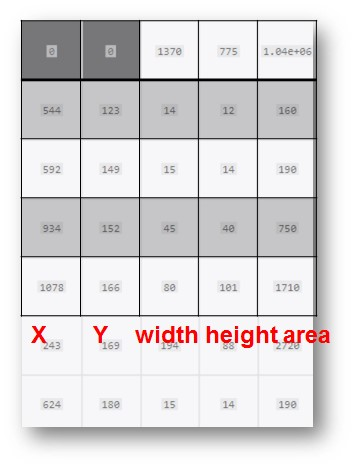## 5.1什么是矩（moment）数学定义：实函数相对于值c的n阶矩为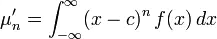从上述公式可以看到，它就是一个加了权重的积分，而权重是(x-c)n，其中n是阶数（n阶矩），如果把它想成一个平面直角系中，c是x轴上的一点，(x-c)n是个x点相对于c点值的n次方。以下是个积分的图示，只要想象一下，它的每个小方块再乘上权重：(xi-c)^n即可得到矩。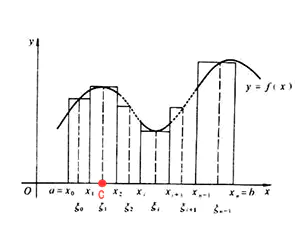轮廓处理中用到的矩，是它在统计学中的应用。以上公式是一元的情况，扩展到图片所在的二元，想象我们有一个图像矩阵，经过了寻找边缘，转换轮廓之后，矩阵中每个值点f(x,y)的值或为0（不是轮廓点），或为1（是轮廓点），当f(x,y)为0时，该积分项也为0，可以不计算，因此，对我们有意义的只有f(x,y)=1的n个点，即轮廓点。在后面公式中记为I(x,y)，x,y为其在图中的坐标，c点扩展到二元，可以视为轮廓的中心点，我们求得的所谓n阶中心矩，就如上述公式所示，积分的权重是轮廓上各点相对于中心位置c的n次方。此时我们可以得到一些统计规律，比如：轮廓边界长度（零阶矩），x/y方向上的均值（即质心，由一阶矩求得），方差（由二阶中心矩求得）,形状特性（Hu矩）5.2. 常用的矩1) 空间矩（spatial moment）i. 用途最简单地轮廓比较，只能用于对比位置，大小，角度完全一致的轮廓。一般来说轮廓矩代表了一条轮廓、一幅图像、一组点集的某些高级特征。ii. 公式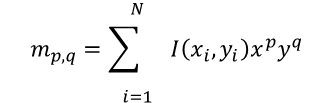在上式中，mp,q代表对象中所有像素的总和，其中每个像素x, y的像素值都乘以因子 xpyq。. 在m00时，这个因子等于1。因此若图像为二值图（如，所有像素都等于0或者1），则 m00代表图像上所有值非零的区域。 当处理轮廓时，结果是轮廓的长度。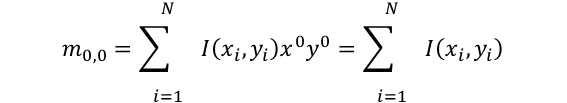mpq表示图像的（p+q）阶矩，一般计算所有3阶的矩（p+q<=3）。其中 I(x,y) 是象素点 (x, y) 的值，一般是1，n是轮廓上点的个数，p和q分别是x维度和y维度上的矩，即m00,m10,m01…m03。零阶矩m00是轮廓上点的简单累加，即轮廓上有多少个点 。一阶矩m10，m01分别是x和y方向上的累加2) 中心矩（central moment）i. 用途xavg和yavg由一阶矩和零阶矩的比值算出（见公式），它是重心坐标，中心矩即是根据x,y与重心的相对位置求取的矩，它使得结果与图像相对于x,y轴的位置无关（与平移无关）。ii. 公式将m10和m01分别除以 m00，能得到整个对象的平均x值和y值。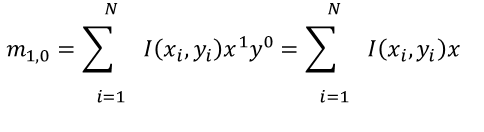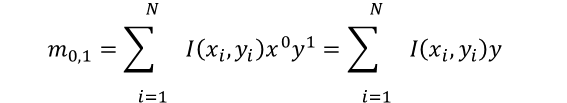中心矩常用μp, q标注，定义如下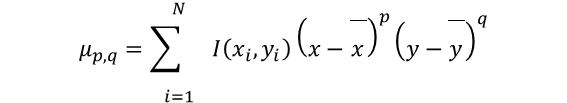其中：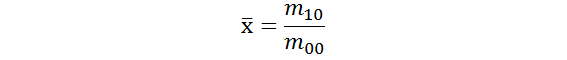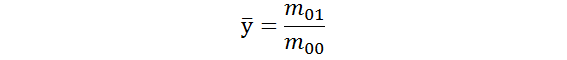3) 归一化的中心矩i. 用途使用m00的幂对中心矩归一化，使得结果与图像大小无关ii. 公式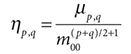4) Hu不变矩i. 用途Hu矩是归一化中心矩的线性组合，它对于缩放，旋转，镜像映射具有不变性。ii. 公式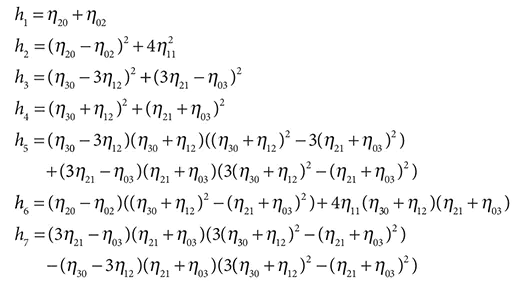最后，我想补充一下我认为适合轮廓分析的场合：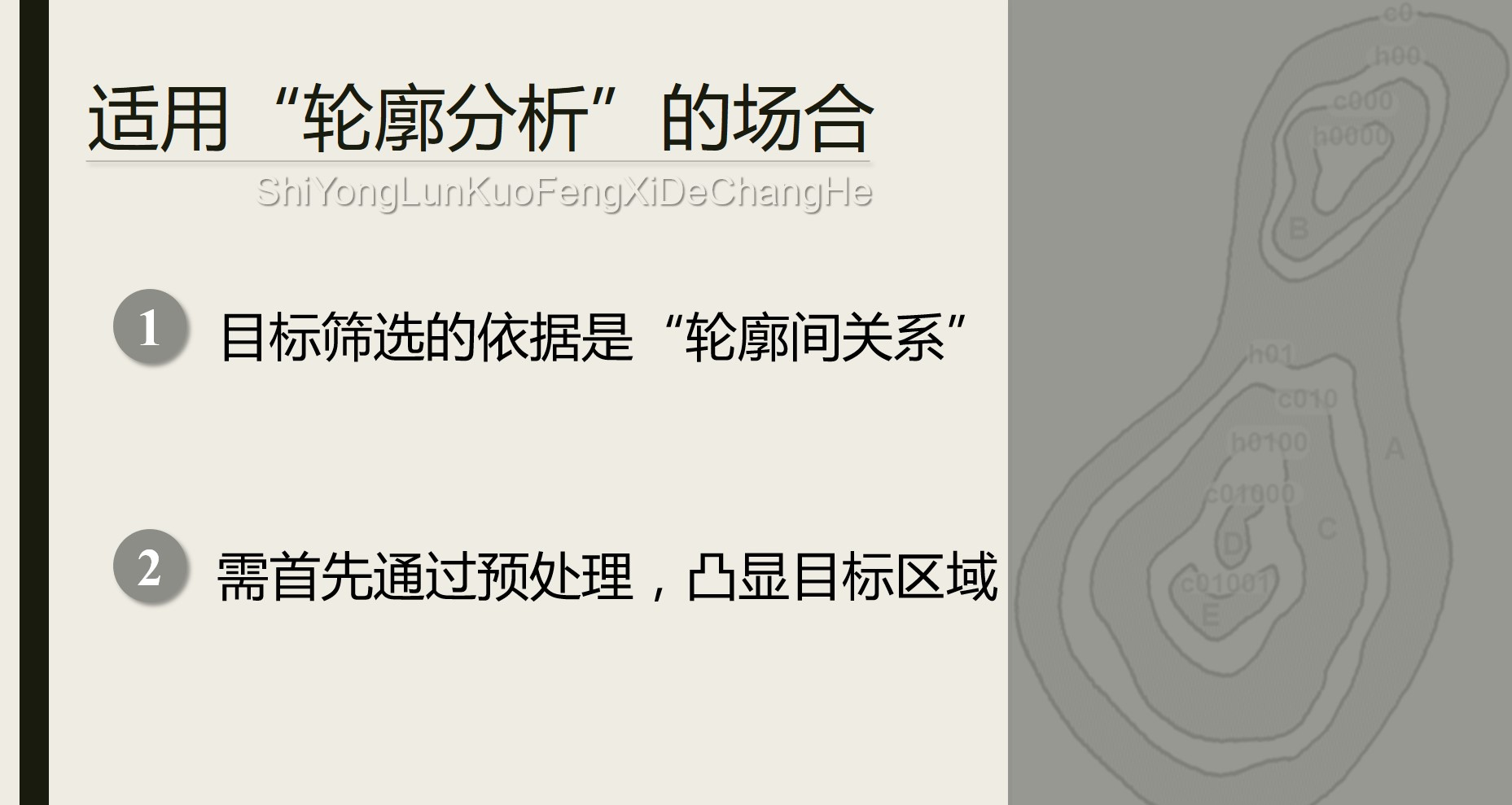posted @ 2020-07-03 06:59  jsxyhelu  阅读(1496)  评论(0编辑  收藏  举报